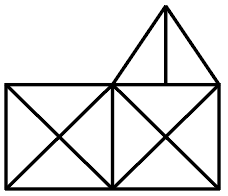Question 13

# How many triangles are there in the following figure.Solution

If figure is in shape of square and two diagonals are there then total numer of triangles is always 8

So, ABCD and CBFG have 8 and 8 triangles means total 16 triangles.

Now, Remaining triangles = BIJ, IJF, BJF, DBG, ACF

Total number of triangles = 8 + 8 + 5 = 21.

• Free SSC Study Material - 18000 Questions
• 230+ SSC previous papers with solutions PDF